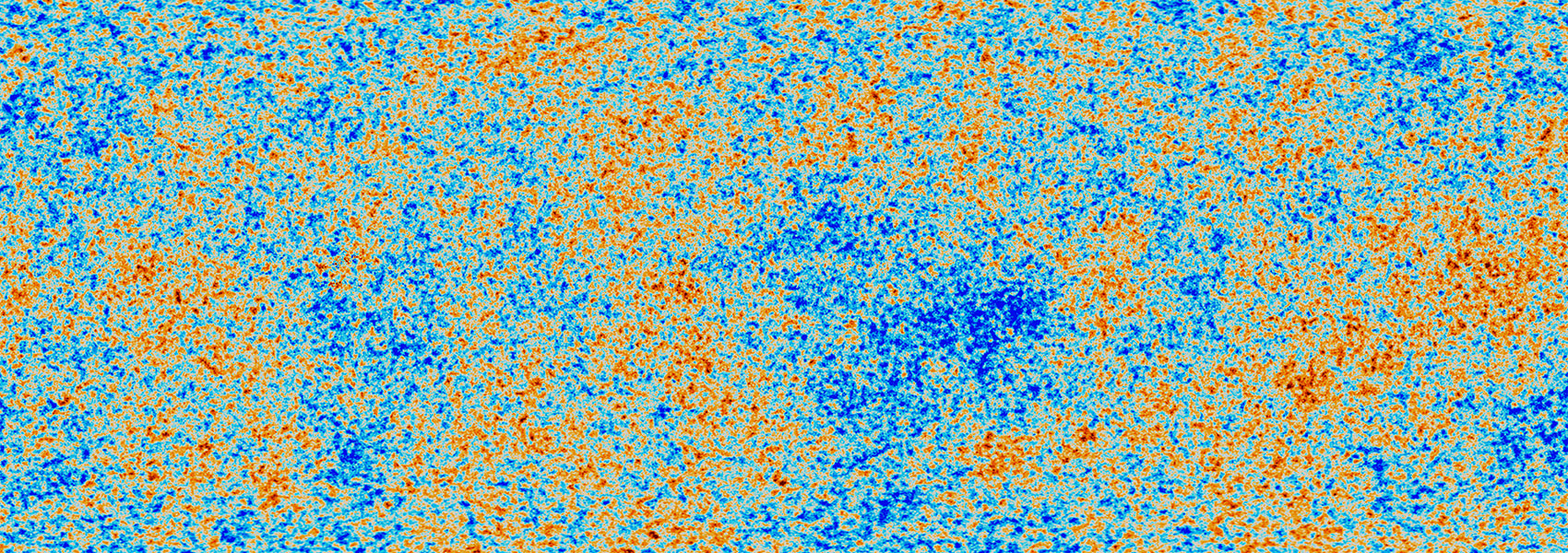# TOI-481 b and TOI-892 b: Two Long-period Hot Jupiters from the Transiting Exoplanet Survey Satellite

November 2020 • 2020AJ....160..235B

Authors • Brahm, Rafael • Nielsen, Louise D. • Wittenmyer, Robert A. • Wang, Songhu • Rodriguez, Joseph E. • Espinoza, Néstor • Jones, Matías I. • Jordán, Andrés • Henning, Thomas • Hobson, Melissa • Kossakowski, Diana • Rojas, Felipe • Sarkis, Paula • Schlecker, Martin • Trifonov, Trifon • Shahaf, Sahar • Ricker, George • Vanderspek, Roland • Latham, David W. • Seager, Sara • Winn, Joshua N. • Jenkins, Jon M. • Addison, Brett C. • Bakos, Gáspár Á. • Bhatti, Waqas • Bayliss, Daniel • Berlind, Perry • Bieryla, Allyson • Bouchy, Francois • Bowler, Brendan P. • Briceño, César • Brown, Timothy M. • Bryant, Edward M. • Caldwell, Douglas A. • Charbonneau, David • Collins, Karen A. • Davis, Allen B. • Esquerdo, Gilbert A. • Fulton, Benjamin J. • Guerrero, Natalia M. • Henze, Christopher E. • Hogan, Aleisha • Horner, Jonathan • Huang, Chelsea X. • Irwin, Jonathan • Kane, Stephen R. • Kielkopf, John • Mann, Andrew W. • Mazeh, Tsevi • McCormac, James • McCully, Curtis • Mengel, Matthew W. • Mireles, Ismael • Okumura, Jack • Plavchan, Peter • Quinn, Samuel N. • Rabus, Markus • Saesen, Sophie • Schlieder, Joshua E. • Segransan, Damien • Shiao, Bernie • Shporer, Avi • Siverd, Robert J. • Stassun, Keivan G. • Suc, Vincent • Tan, Thiam-Guan • Torres, Pascal • Tinney, Chris G. • Udry, Stephane • Vanzi, Leonardo • Vezie, Michael • Vines, Jose I. • Vuckovic, Maja • Wright, Duncan J. • Yahalomi, Daniel A. • Zapata, Abner • Zhang, Hui • Ziegler, Carl

Abstract • We present the discovery of two new 10 day period giant planets from the Transiting Exoplanet Survey Satellite mission, whose masses were precisely determined using a wide diversity of ground-based facilities. TOI-481 b and TOI-892 b have similar radii (0.99 ± 0.01 ${R}_{{\rm{J}}}$ and 1.07 ± 0.02 ${R}_{{\rm{J}}}$ , respectively), and orbital periods (10.3311 days and 10.6266 days, respectively), but significantly different masses (1.53 ± 0.03 ${M}_{{\rm{J}}}$ versus 0.95 ± 0.07 ${M}_{{\rm{J}}}$ , respectively). Both planets orbit metal-rich stars ( $[\mathrm{Fe}/{\rm{H}}]$ = $+0.26\pm 0.05$ dex and $[\mathrm{Fe}/{\rm{H}}]$ = $+0.24\pm 0.05$ for TOI-481 and TOI-892, respectively) but at different evolutionary stages. TOI-481 is a ${M}_{\star }$ = 1.14 ± 0.02 ${M}_{\odot }$ , ${R}_{\star }$ = 1.66 ± 0.02 ${R}_{\odot }$ G-type star ( ${T}_{\mathrm{eff}}$ = $5735\pm 72$ K), that with an age of 6.7 Gyr, is in the turn-off point of the main sequence. TOI-892 on the other hand, is a F-type dwarf star ( ${T}_{\mathrm{eff}}$ = $6261\pm 80$ K), which has a mass of ${M}_{\star }$ = 1.28 ± 0.03 ${M}_{\odot }$ and a radius of ${R}_{\star }$ = 1.39 ± 0.02 ${R}_{\odot }$ . TOI-481 b and TOI-892 b join the scarcely populated region of transiting gas giants with orbital periods longer than 10 days, which is important to constrain theories of the formation and structure of hot Jupiters.